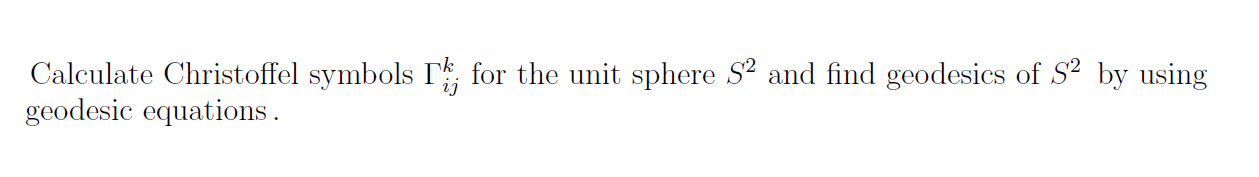# Question Solved1 AnswerCalculate Christoffel symbols Ti , for the unit sphere S2 and find geodesics of $2 by using geodesic equations.VFSIUN The Asker · AlgebraTranscribed Image Text: Calculate Christoffel symbols Ti , for the unit sphere S2 and find geodesics of$2 by using geodesic equations.
More
Transcribed Image Text: Calculate Christoffel symbols Ti , for the unit sphere S2 and find geodesics of \$2 by using geodesic equations.